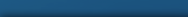Increasing a web page’s PageRank is most easily done by increasing the number of inbound links to the page. PageRank is a complex system that has at its heart the following algorithm:

PR(A) = (1-d) + d (PR(T1)/C(T1) + … + PR(Tn)/C(Tn))

Here, d is a parameter that determines the relevance of a given page, X. In order to investigate how the PageRank algorithm works dynamically, we will assume that the PageRank will increase by an amount, Δr, given by:

Δr = d x PR(X) / C(X)

. . . Where C(X) is the total number of outbound links, PR(X) is the PageRank of page Xʼ, and d, as mentioned above, is a parameter determining the reelvance of a given page. This alone gives us a lot to work with, and allows us to come to some important conclusions. First, the change in PageRank scales directly with the PageRank of the linking page X and inversely with the number of outbound links of page X. Clearly, then, it’s important to increase the number of quality links to your page if you want to increase its PageRank, where “quality” is here measured as a function of the PageRank of the linking page.

Something else to consider is the fact that, if a page Y links to other pages that link back to it, page Y will earn an additional PageRank benefit from the additional inbound links. This is a clever and natural way to do your page some good. Let’s take a look at an example to see what effects singular inbound links have on PageRank.

Assume we have a website with four pages – A, B, C, and D, which link to one another cyclically (i.e., A links to B, B links to C, etc.). For now, it’s best to ignore the effects of external links from other websites, since that just complicates things and we’re just trying to understand the basics. Ignoring external factors, then, we can see that each page has a Page Rank of 1.

Now, let’s add another page, Z, to the mix. We’ll assume PR(Z) = 10, and Z links to A as its only outbound link. Setting d = 0.5, we have the following set of equations:

PR(A) = 0.5 + 0.5 (PR(X) + PR(D)) = 5.5 + 0.5 PR(D)

PR(B) = 0.5 + 0.5 PR(A)

PR(C) = 0.5 + 0.5 PR(B)

PR(D) = 0.5 + 0.5 PR(C)

Solving yields:

PR(A) = 19/3 = 6.33

PR(B) = 11/3 = 3.67

PR(C) = 7/3 = 2.33

PR(D) = 5/3 = 1.67

What can we conclude from this? Well, the most significant conclusion is the fact that the effect of A, with an increment Δr = 5, is passed on and “filtered” by each page in the cycle.

## Damping Factor

The damping factor is a crucial parameter. The damping factor effectively allows us to see how much a page’s PageRank is propagated through its outbound links. Changing the damping factor d allows us to see just how this works. In the above examples, if we set d = 0.75, we get the following solutions to the above equations:

PR(A) = 419/35 = 11.97

PR(B) = 323/35 = 9.23

PR(C) = 251/35 = 7.17

PR(D) = 197/35 = 5.63

. . . Whence it is clear that PR(A) propagated more strongly in the latter. This is not the only change, however. If we run the calculation for PR(A), we’ll find PR(A) = 7.5, so we see that the propagation is stronger, and the initial PageRank is of higher magnitude, as well.The takeway? The higher the damping factor, the higher the degree of propagation, and the better distributed the PageRank is over the page’s outbound links.

Now let’s complicate things a little bit and look at additional links. If we set d = 0.5 again, we’ll find a gross Pagerank of:

ΣPR(i) = 14

This is significant, because the PageRank of each page (A, B, C, D) without the link from Z is 1, giving a sum of 4. The addition of the link to Z adds 10 to the gross PageRank. With a damping factor of d  = 0.75, we have a gross PageRank of 34, making the significance of the damping factor even more obvious. The differential for accumulated PageRanks is given by a relatively easy formula:

(d / (1-d)) × (PR(X) / C(X))

. . . Where X is a page linking out, PR(X) is PageRank of X, and C(X) is the number of outbound links from X. This formula is valid only for additional links that point to a page within a closed system, or cycle. An example of such a system is a website that links to no external sites. This is, of course, a simplification, but it’s a good start to understanding how things work in the real world.

## PageRank-1 Rule

The PageRank-1 rule illustrates one of the most important principles of good SEO practice. The PageRank algorithm presented above might not be Google’s precisely, but it does work in many of the areas a good model would be expected to. In particular, it satistifes PageRank-1, which is the statement that the absolute number of inbound links is not important – it’s the quality of the links that counts. In other words, haveing 200 inbound links is useless, if all of those links are from low-quality, low-traffic sites. Having a single inbound from, say, Wired, on the other hand, is the perfect way to ensure a high and increasing PageRank. The PageRank-1 rule is satistfied by the above model, and is a fact of Google’s own ranking algorithms.

Here, we’ve taken a look at an extremely accurate model to better understand the functionality of Google’s algorithms so that we can better take advantage of techniques to increase PageRank.

### Related Articles